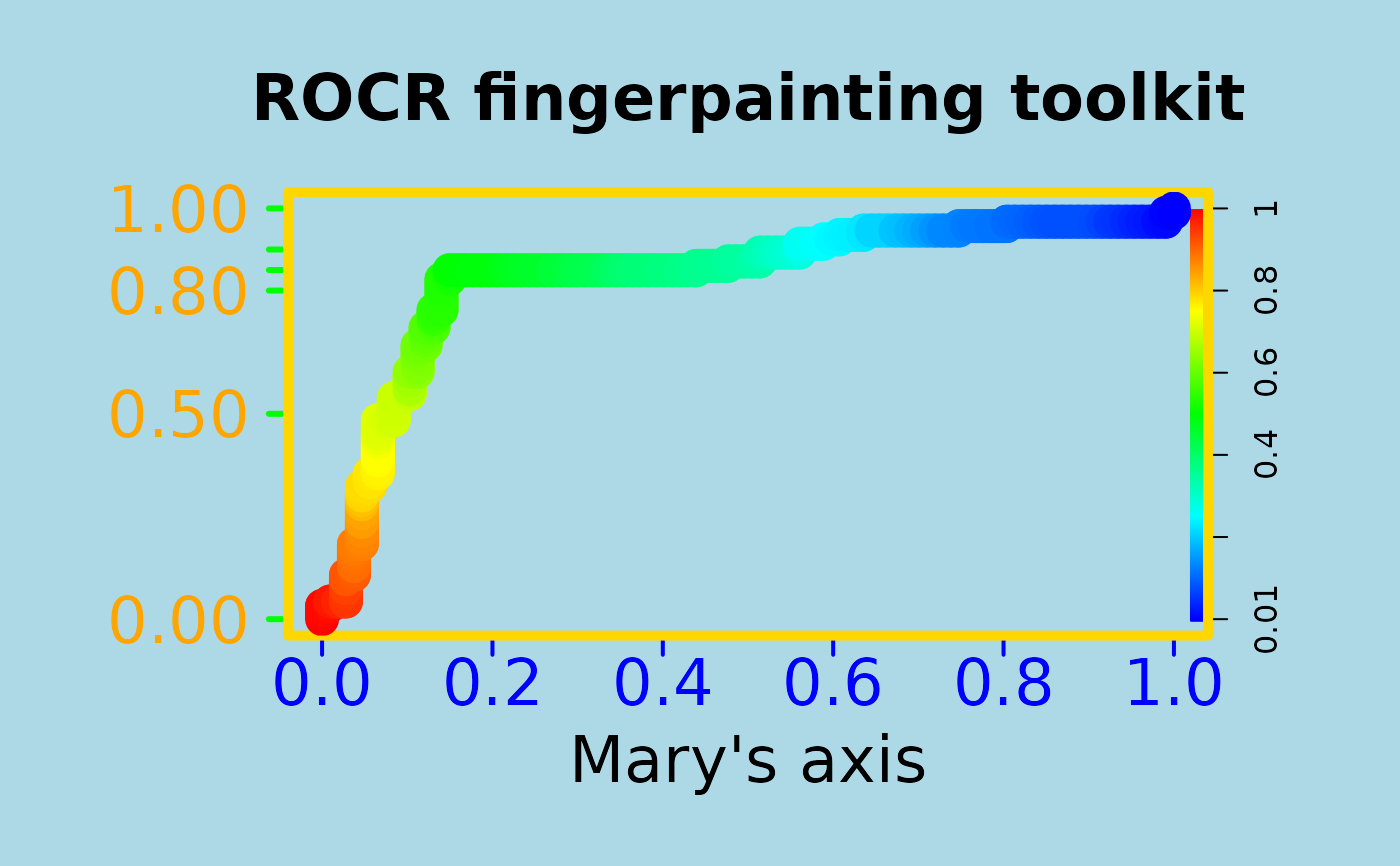This is the method to plot all objects of class performance.

# S4 method for performance,missing
plot(
x,
y,
...,
avg = "none",
spread.estimate = "none",
spread.scale = 1,
show.spread.at = c(),
colorize = FALSE,
colorize.palette = rev(rainbow(256, start = 0, end = 4/6)),
colorkey = colorize,
colorkey.relwidth = 0.25,
colorkey.pos = "right",
print.cutoffs.at = c(),
cutoff.label.function = function(x) {     round(x, 2) },
downsampling = 0,
add = FALSE
)

# S3 method for performance
plot(...)

## Arguments

x an object of class performance not used Optional graphical parameters to adjust different components of the performance plot. Parameters are directed to their target component by prefixing them with the name of the component (component.parameter, e.g. text.cex). The following components are available: xaxis, yaxis, coloraxis, box (around the plotting region), points, text, plotCI (error bars), boxplot. The names of these components are influenced by the R functions that are used to create them. Thus, par(component) can be used to see which parameters are available for a given component (with the expection of the three axes; use par(axis) here). To adjust the canvas or the performance curve(s), the standard plot parameters can be used without any prefix. If the performance object describes several curves (from cross-validation runs or bootstrap evaluations of one particular method), the curves from each of the runs can be averaged. Allowed values are none (plot all curves separately), horizontal (horizontal averaging), vertical (vertical averaging), and threshold (threshold (=cutoff) averaging). Note that while threshold averaging is always feasible, vertical and horizontal averaging are not well-defined if the graph cannot be represented as a function x->y and y->x, respectively. When curve averaging is enabled, the variation around the average curve can be visualized as standard error bars (stderror), standard deviation bars (stddev), or by using box plots (boxplot). Note that the function plotCI, which is used internally by ROCR to draw error bars, might raise a warning if the spread of the curves at certain positions is 0. For stderror or stddev, this is a scalar factor to be multiplied with the length of the standard error/deviation bar. For example, under normal assumptions, spread.scale=2 can be used to get approximate 95% confidence intervals. For vertical averaging, this vector determines the x positions for which the spread estimates should be visualized. In contrast, for horizontal and threshold averaging, the y positions and cutoffs are determined, respectively. By default, spread estimates are shown at 11 equally spaced positions. This logical determines whether the curve(s) should be colorized according to cutoff. If curve colorizing is enabled, this determines the color palette onto which the cutoff range is mapped. If true, a color key is drawn into the 4% border region (default of par(xaxs) and par(yaxs)) of the plot. The color key visualizes the mapping from cutoffs to colors. Scalar between 0 and 1 that determines the fraction of the 4% border region that is occupied by the colorkey. Determines if the colorkey is drawn vertically at the right side, or horizontally at the top of the plot. This vector specifies the cutoffs which should be printed as text along the curve at the corresponding curve positions. By default, cutoff annotations along the curve or at the color key are rounded to two decimal places before printing. Using a custom cutoff.label.function, any other transformation can be performed on the cutoffs instead (e.g. rounding with different precision or taking the logarithm). ROCR can efficiently compute most performance measures even for data sets with millions of elements. However, plotting of large data sets can be slow and lead to PS/PDF documents of considerable size. In that case, performance curves that are indistinguishable from the original can be obtained by using only a fraction of the computed performance values. Values for downsampling between 0 and 1 indicate the fraction of the original data set size to which the performance object should be downsampled, integers above 1 are interpreted as the actual number of performance values to which the curve(s) should be downsampled. If TRUE, the curve(s) is/are added to an already existing plot; otherwise a new plot is drawn.

A detailed list of references can be found on the ROCR homepage at http://rocr.bioinf.mpi-sb.mpg.de.

## See also

prediction, performance, prediction-class, performance-class

## Author

Tobias Sing tobias.sing@gmail.com, Oliver Sander osander@gmail.com

## Examples

# plotting a ROC curve:
library(ROCR)
data(ROCR.simple)
pred <- prediction( ROCR.simple$predictions, ROCR.simple$labels )
pred
#> A prediction instance
#>   with 200 data pointsperf <- performance( pred, "tpr", "fpr" )
perf
#> A performance instance
#>   'False positive rate' vs. 'True positive rate' (alpha: 'Cutoff')
#>   with 201 data pointsplot( perf )# To entertain your children, make your plots nicer
# using ROCR's flexible parameter passing mechanisms
# (much cheaper than a finger painting set)
par(bg="lightblue", mai=c(1.2,1.5,1,1))
plot(perf, main="ROCR fingerpainting toolkit", colorize=TRUE,
xlab="Mary's axis", ylab="", box.lty=7, box.lwd=5,
box.col="gold", lwd=17, colorkey.relwidth=0.5, xaxis.cex.axis=2,
xaxis.col='blue', xaxis.col.axis="blue", yaxis.col='green', yaxis.cex.axis=2,
yaxis.at=c(0,0.5,0.8,0.85,0.9,1), yaxis.las=1, xaxis.lwd=2, yaxis.lwd=3,
yaxis.col.axis="orange", cex.lab=2, cex.main=2)